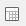# Calculating median scores

The following example uses the Crocodile Rock Cafe Survey. Question 6a is an attitude questions using a rating scale of very good to very poor coded 1 to 5.

1. Clickto display the Analysis Definition dialog box.
2. Specify the Analysis as Q6e, Choice of food.
3. Specify the Break as Q11, Age range.
4. Click on the Summary Statistics tab.
5. Highlight Median from the ‘Available’ list and click > to add it to the Used column. You can position the Median scores above the body of the table by using the Move Up button.
6. Click OK to display the table.

Remember that calculating the median means finding the mid-point. In this example 65 respondents are 25-34; therefore the mid-point is 32. The 32nd respondent in that age group responded ‘Good’ so the median is ‘Good’. 72 respondents are 35-44 and the mid-point is 36. The 36th respondent comes within the responses of ‘OK’ and the median is therefore ‘OK’.

You can add a score to the table by checking the Scored box. The median will be calculated using the score displayed.

Contents